# Irreversibility analysis for a mixed convective flow of a reactive couple stress fluid flow through channel saturated porous materials

Irreversibility analysis for a mixed convective flow of a reactive couple stress fluid flow through channel saturated porous materials

Department of Mathematical Sciences, University of South Africa, Pretoria 003, South Africa.

Department of Mathematics, Tai Solarin University of Education, Ijagun, Nigeria

Department of Mathematical Sciences, Redeemer’s University, Ede, Nigeria

Department of Mathematics, Vaal University of Technology, Vanderbijlpark 1911, South Africa.

Department of Physical Sciences, Redeemer’s University, Ede, Nigeria

Corresponding Author Email:
Page:
633-638
|
DOI:
https://doi.org/10.18280/ijht.350321
|
Accepted:
|
Published:
30 September 2017
| Citation

OPEN ACCESS

Abstract:

The present work examines the eﬀect of temperature-dependent fluid density on the reactive couple stress fluid flowing steadily in a vertical channel saturated with porous materials. The thermal effect gave rise to a linked differential system of equations. In the dimensionless form, the boundary-valued problems (BVP) are uncoupled and solved by using Adomian decomposition method (ADM). The solutions are used to obtain expressions for entropy generation and Bejan heat irreversibility number in the porous medium. The relevant fluid parameters are varied and discussed physically. The present result shows good agreement with the previously obtained result when buoyancy force is neglected. The present study is relevantal in the thermal treatment of combustible fluids in chemical and petro-chemical engineering.

Keywords:

reactive fluid, couple stress fuid, porous medium, buoyancy eﬀect and adomian decomposition method (ADM)

1. Introduction

In recent times, studies involving fluid flow with convective heat transfer through parallel plates have attracted huge consideration because of its importance in many engineering and industrial processes. Several researchers [1-5] (and references therein) have investigated several aspects of the convective flow problems under different flow conditions. However, all the studies above are limited to the Newtonian case in which the classical Navier-Stokes equation is valid. In reality, most fluid of mechanical, medicinal and industrial significance contain several additives in the form of tiny microstructure like polymer additives in synthetic fluids, red blood cells in blood, drug particles in doping by athletes, targeted drug delivery in medicine and lots more. As shown in , Kaladhar et al., described the forced convective flow of reactive couple stress fluids undergoing destructive chemical reaction. Adesanya et al.  reported the inherent irreversibility in the couple stress fluid flow through horizontal channel. Asad et al. , highlighted the significance of the flow and heat transfer of couple stress fluid having temperature-dependent thermal conductivity. Nayak et al.,  reported the hydromagnetic couple stress fluid flow and heat transfer in a barrier-filled rotating channel. Zelalem et al.,  discussed the fluid flow and heat transfer of couple stress fluid through parallel walls endowed with extended porous materials. Interested readers can see [11-16] for more significant results on couple stress fluid flow.

Motivated by the study in , the primary concern here is to investigate the impact of buoyancy force on the entropy generation due to convective flow and heat transfer. To the best our understanding, the flow situations reported here has not been conducted in the literature. The study is necessary due to the strong exothermic nature of some reactive fluid. Hence, a more accurate approximation would be achieved if the variation of fluid density with temperature is taken into consideration. The next section describes the flow assumptions in detail with the mathematical analysis. In section three, the Adomian method of solution will be presented. Section four of the work describes the tabular and graphical results while section five gives the summary of the work.

2. Mathematical Formulation

Consider the steady flow of an hydrodynamically and thermally developed combustible couple stress fluid through a vertical channel of distance h apart. The channel walls are maintained isothermally at a constant temperature T0. As shown in igure 1 below, the no-slip and the stress free conditions are imposed on the channel walls. The constant pressure gradient is in the direction of the flow and variation in density due to the presence of buoyancy force eﬀect is taken into account in the momentum equation.

## 1.png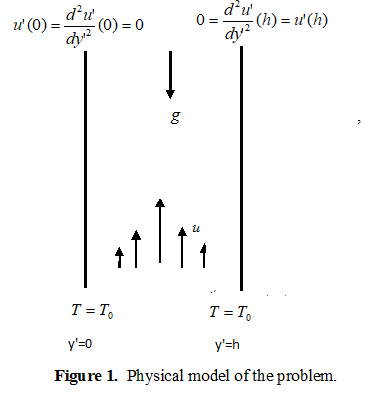Therefore, the linearized governing momentum and energy equations following the Boussinesq approximation can be written as :

$0=-\frac{d P}{d x}+\mu \frac{d^{2} u^{\prime}}{d y^{\prime 2}}-\frac{\mu u^{\prime}}{K}-\eta \frac{d^{4} u^{\prime}}{d y^{4}}+\rho g \alpha\left(T-T_{0}\right)$     (1)

$0=\frac{d^{2} T}{d y^{\prime 2}}+\frac{Q C_{0} A}{k} e^{-\frac{E}{R T}}+\frac{\mu}{k}\left(\frac{d u^{\prime}}{d y^{\prime}}\right)^{2}$

$+\frac{\mu u^{2}}{k K}+\frac{\eta}{k}\left(\frac{d^{2} u^{\prime}}{d y^{2}}\right)^{2}$     (2)

The last term in (1) is due to the effect of buoyancy force as in [1-5] together with the suitable boundary conditions at the plate ends are given to be:

$T(0)=T(h)=T_{0}$

$u^{\prime}(0)=\frac{d^{2} u^{\prime}}{d y^{\prime 2}}(0)=0=\frac{d^{2} u^{\prime}}{d y^{\prime 2}}(h)=u^{\prime}(h)$     (3)

Also, the entropy generation rate in the couple stress fluid flow can be represented with this expression as given by  as:

$E_{G}=\frac{k}{T_{0}^{2}}\left(\frac{d T}{d y^{\prime}}\right)^{2}+\frac{\mu}{T_{0}}\left(\frac{d u^{\prime}}{d y^{\prime}}\right)^{2}+\frac{\mu u^{\prime 2}}{T_{0} K}+\frac{\eta}{T_{0}}\left(\frac{d^{2} u^{\prime}}{d y^{2}}\right)^{2}$    (4)

With the under-listed non-dimensional quantities:

$y=\frac{y^{\prime}}{h}, y=\frac{u^{\prime}}{U M}, \theta=\frac{E\left(T-T_{0}\right)}{R T_{0}^{2}}, M=\frac{h^{2}}{\mu U} \frac{d P}{d x}, \gamma=\frac{h}{l}$

$\varepsilon=\frac{R T_{0}}{E}, \lambda=\frac{Q E A C_{0} h^{2}}{R T_{0}^{2} k} e^{-\frac{E}{R T_{0}}}, \delta=\frac{U^{2} \mu M^{2}}{Q A C_{0} h^{2}} e^{\frac{E}{R T_{0}}}, l=\sqrt{\frac{\eta}{\mu}}$

$\beta=\sqrt{\frac{1}{D a}}, D a=\frac{K}{h^{2}}, G_{r}=\frac{\rho g \alpha R T_{0}^{2} h^{2}}{M U \mu E}, N_{s}=\frac{h^{2} E^{2} E_{G}}{k R^{2} T_{0}^{2}}$    (5)

we procure the under--listed boundary valued problems regulating the fluid flow from equations (1 – 4) as:

$1+\frac{d^{2} u}{d y^{2}}-\beta^{2} u-\frac{1}{\gamma} \frac{d^{4} u}{d y^{4}}+G_{r} \theta=0$     (6)

$\frac{d^{2} \theta}{d y^{2}}+\lambda\left(e^{\frac{\theta}{1+\varepsilon \theta}}+\delta\left[\left(\frac{d u}{d y}\right)^{2}+\frac{1}{\gamma}\left(\frac{d^{2} u}{d y^{2}}\right)^{2}+\beta^{2} u^{2}\right]\right)=0$      (7)

together with the boundary conditions:

\begin{aligned} \theta(0) &=\theta(1)=0 \\ u(0) &=\frac{d^{2} u}{d y^{2}}(0)=0=\frac{d^{2} u}{d y^{2}}(1)=u(1) \end{aligned}     (8)

and the entropy generation rate in dimensionless form is:

$N_{s}=\left(\frac{d \theta}{d y}\right)^{2}+\frac{\delta \lambda}{\varepsilon}\left(\left(\frac{d u}{d y}\right)^{2}+\frac{1}{\gamma}\left(\frac{d^{2} u}{d y^{2}}\right)^{2}+\beta^{2} u^{2}\right)$      (9)

Furthermore, let N1 be $\left(\frac{d \theta}{d y}\right)^{2}$ which represents the irreversibility due to heat transfer.

Also, let N2b he $\frac{\delta \lambda}{\varepsilon}\left(\left(\frac{d u}{d y}\right)^{2}+\frac{1}{\gamma}\left(\frac{d^{2} u}{d y^{2}}\right)^{2}+\beta^{2} u^{2}\right)$which stands for the entropy generation due to the combined effects of the viscous dissipation and porous permeability. Then, Bejan number (Be) is defined as the heat irreversibility ratio as follows:

$B e=\frac{N_{1}}{N_{s}}=\frac{1}{1+\Phi}$ where $\Phi=\frac{N_{2}}{N_{1}}$     (10)

The coupled nonlinear equations for momentum in (6) and energy in (7) with the boundary conditions in (8) are solved by making use of ADM [17-19]. First, the BVP are converted to the integral forms as follows:

$u(y)=a_{0} y^{3}+b_{0} y+\frac{\gamma y^{4}}{24}$$+\gamma \int_{0}^{y} \int_{0}^{y} \int_{0}^{y} \int_{0}^{y}$$\left[\frac{d^{2} u}{d Y^{2}}-\beta^{2} u+G_{r} \theta\right] d Y d Y d Y d Y$    (11)

$\theta(y)=c_{0} y-\lambda \int_{0}^{y} \int_{0}^{y}\left(e^{\frac{\theta}{1+s \theta}}+\delta\left[\left(\frac{d u}{d Y}\right)^{2}+\frac{1}{\gamma}\left(\frac{d^{2} u}{d Y^{2}}\right)^{2}+\beta^{2} u^{2}\right]\right) d Y d Y$    (12)

where a0,, b0 and c0 are constants that will be determined by employing the appropriate requirements stated in (8). By ADM, we take an infine series solutions of the form:

$u(y)=\sum_{n=0}^{\infty} u_{n}(Y)$ and $\theta(y)=\sum_{n=0}^{\infty} \theta_{n}(Y)$     (13)

substituting (13) into the coupled equations (11) and (12) to have

$\sum_{n=0}^{\infty} u_{n}(Y)=a_{0} y^{3}+b_{0} y+\frac{\gamma y^{4}}{24}+$$\gamma \int_{0}^{y} \int_{0}^{y} \int_{0}^{y} \int_{0}^{y}\left[\frac{d^{2}}{d Y^{2}}\left(\sum_{n=0}^{\infty} u_{n}(Y)\right)-\beta^{2}\left(\sum_{n=0}^{\infty} u_{n} 0\right)\right] d y Y d Y d Y d Y$$+\gamma \int_{0}^{y} \int_{0}^{y} \int_{0}^{y} \int_{0}^{y}$$\left[G_{r}\left(\sum_{n=0}^{\infty} \theta_{n}(Y)\right)\right] d y Y d Y d Y d Y (14) and \sum_{n=0}^{\infty} \theta_{n}(y)=c_{0} y-\lambda \int_{0}^{y} \int_{0}^{y}$$\left(\begin{array}{c}{\sum_{n=0}^{\infty} \theta_{n}(y)} \\ {e^{1+\varepsilon \sum_{n=0}^{\infty} \theta_{n}(y)}}\end{array}\right.$$+\delta\left[\left(\frac{d}{d Y}\left(\sum_{n=0}^{\infty} u_{n}(Y)\right)\right)^{2}\right] d Y d Y$$-\lambda \int_{0}^{y} \int_{0}^{y}$$\left(\delta\left[\frac{1}{\gamma}\left(\frac{d^{2}}{d Y^{2}}\left(\sum_{n=0}^{\infty} u_{n}(Y)\right)\right)^{2}\right.\right.$$+\beta^{2}\left(\sum_{n=0}^{\infty} u_{n}(Y)\right)^{2} ] d Y d Y$     (15)

let the nonlinear terms in (15) be represented by:

$\sum_{n=0}^{\infty} A_{n}(y)=e^{\frac{\sum_{n=0}^{\infty} \theta_{n}(y)}{1+\varepsilon \sum_{n=0}^{\infty} \theta_{n}(y)}}$

$\sum_{n=0}^{\infty} B_{n}(y)=\left[\left(\frac{d}{d Y}\left(\sum_{n=0}^{\infty} u_{n}(y)\right)\right)^{2} \frac{1}{\gamma}\left(\frac{d^{2}}{d Y^{2}}\left(\sum_{n=0}^{\infty} u_{n}(y)\right)\right)^{2}\right]$$+\left[\beta^{2}\left(\left(\sum_{n=0}^{\infty} u_{n}(y)\right)\right)^{2}\right]$    (16)

The Adomial polynomials can be obtained by the Taylor’s expansion of the series in (16). Then the zeroth order approximation becomes:

$u_{0}(y)=a_{0} y^{3}+b_{0} y+\frac{\gamma y^{4}}{24}$

$\theta_{n}(y)=0$     (17)

While other terms of the series can be obtained by using the relations:

\begin{aligned} u_{n+1}(y) &=\gamma \int_{0}^{y} \int_{0}^{y} \int_{0}^{y}\left[\frac{d^{2} u_{n}}{d Y^{2}}-\beta^{2} u_{n}+G_{r} \theta_{n}\right] d Y d Y d Y d Y \\ \theta_{n+1}(y) &=c_{0} y-\lambda \int_{0}^{y} \int_{0}^{y}\left(A_{n}+\delta B_{n}\right) d Y d Y \end{aligned}     (18)

such that the approximate solution of the coupled BVP can be obtained in the form of the partial sums:

$u(y)=\sum_{n=0}^{k} u_{n}(Y)$ and $\theta(y)=\sum_{n=0}^{k} \theta_{n}(Y)$     (19)

The partial sums in (19) are then utilized in (9)-(10) to compute the entropy generation and the Bejan profiles provided in the following section. For easy iteration of the tedious calculations, equations (9)-(10), (16)-(19) are programmed in the Mathematica software package.

4. Results and Discussions

In this section, the velocity, temperature, entropy generation and heat irreversibility ratio profiles are presented. In Table 02, the rapid convergence of the Adomian series solution to the couple problem is presented. It is interesting to note that, convergence of the series is obtained with just few iterations. Also, the validation of the present result in the absence of Grashof number is presented in Table 02. From the table,a perfect agreement is observed bewteen the exact result presented in  and the the Adomian series approach.

Table 1. Rapid convergence of the series solution

 ε = δ = 0.1, λ = β = 0.2, γ = Gr = 0.5 n a0 b0 c0 0 - 0.250000 0.0208333 0 1 -0.240422 0.0198889 0.100037 2 -0.242108 0.0200432 0.101764 3 -0.242143 0.0200482 0.101755 4 -0.242143 0.0200482 0.101755

Table 2. Validation of numerical results of the velocity profile

 β = 0.1, γ = 1, Gr = 0 u(y) Exact solutions from  ADM Absolute Error 0.0 0. 0 0 0.1 0.00371505 0.00371574 6.86111× 10-6 0.2 0.00702528 0.00702658 1.30462× 10-6 0.3 0.00961357 0.00961536 1.78656× 10-6 0.4 0.01125560 0.01125770 2.12648× 10-6 0.5 0.01181780 0.01182000 2.18983× 10-6 0.6 0.01125560 0.01125770 2.12648× 10-6 0.7 0.00961357 0.00961536 1.78656× 10-6 0.8 0.00702528 0.00702658 1.30462× 10-6 0.9 0.00371505 0.00371574 6.86111× 10-6 1.0 0 -2.45939 × 10-18 2.45939 × 10-18

Figure 2 represents the velocity profile with variations in the Grashof number. From the result, an increase in the Grashof number is seen to enhance the fluid temperature since an increase in fluid temperature increases the thermal expansion of the fluid, and the upthrust force will increase significantly in the vertical channel. Figure 3 shows the influence of couple stress inverse parameter on the fluid flow. As observed from the graphical result, an increase in the couple stress inverse parameter shows a corresponding increase in the flow velocity as a consequence of the shear thinning of the fluid as the couple stress inverse parameter increases. However, as the couple stress inverse parameter decreases, the shear thickening property of the fluid becomes prominent in the flow channel. As revealed in Figure 4, an increase in Grashof number is seen to enhance the temperature profile. The result is correct since Grashof number increases the flow velocity. Hence a rise in the fluid temperature is expected due to volumetric expansion. The result in Figure 5 revealed the effect of couple stress inverse parameter on the fluid temperature distribution within the flow channel. As observed, as the couple stress inverse parameter increases, the fluid becomes thinner, as a result, inter-particle collisions becomes higher, and the fluid temperature rises. Furthermore, since both Grashof number and couple stress inverse parameter enhances both flow and temperature profiles, it is expected to enhance entropy generation within the channel. In the real sense, the couple stress inverse parameter will ultimately minimize entropy generation as it decreases as reported in Figures 6-7. Finally, in Figures 8-9, it is observed that as both Grashof number and couple stress inverse parameter increases, heat irreversibility due to fluid friction dominates over heat transfer irreversibility in the flow channel.

## 2.pngFigure 2. Effect of Gr on u(y)

## 3.pngFigure 3. Effect of γ on u(y)

## 4.png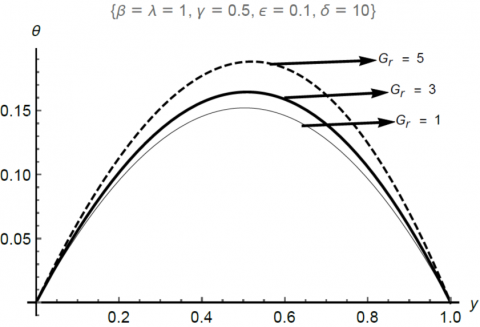Figure 4. Effect of Gr on ϴ(y)

## 5.png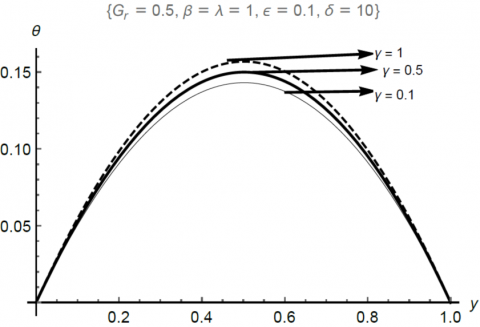Figure 5. Effect of γ on ϴ(y)

## 6.pngFigure 6. Effect of Gr on Ns

## 7.png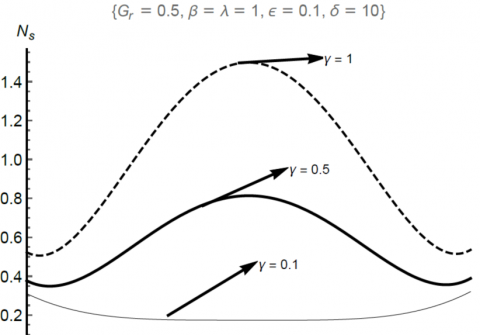Figure 7. Effect of γ on Ns

## 8.pngFigure 8. Effect of Gr on Be

## 9.png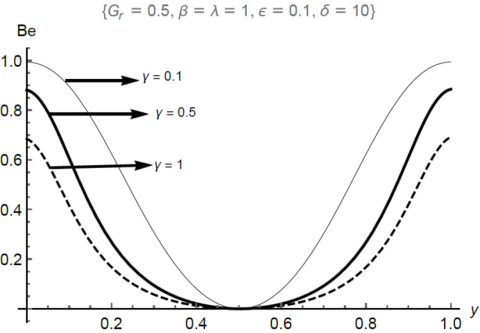Figure 9. Effect of γ on Be

5. Conclusions

The effect of temperature-depend density on the steady flow of a highly combustible couple stress fluid through a channel, due to convective flow and heat transfer, was presented. The nonlinear equations governing the fluid flow were formulated, non-dimensionalized and solved by using Adomian decomposition method. The result of the computations highlights the significance of couple stresses in reducing the fluid temperature when undergoing exothermic chemical reactions and minimizing entropy generation. This is important in improving yields and enhancing the safety of the environment.

Nomenclature
 P Modified fluid pressure K Darcy's permeability constant. g Acceleration due to gravity T0 The wall temperature k Thermal conductivity of the fluid Q Heat of the reaction term C0 Reactant species initial concentration, h The channel width (x,y) Distance measured in the axial and normal direction A Reaction rate constant E Activation energy U Fluid velocity R Universal gas constant U Fluid characteristic velocity l Fluid molecular dimension M Pressure gradient Da Darcy number Gr Grashof number EG Dimensional entropy generation rate Ns Dimensionless entropy generation rate Greek symbols μ Dynamic viscosity coefficient $\eta$ Couple stress coefficient $\rho$ Fluid density $\varepsilon$ Activation energy parameter $\lambda$ Frank—Kamenettski parameter $\gamma$ Couple stress inverse parameter $\delta$ Viscous heating parameter $\beta$ Porous medium permeability parameter $\alpha$ Thermal expansion coefficient $\theta$ Fluid temperature
References

 Kazmierczak M., Chinoda Z. (1992). Buoyancy-driven flow in an enclosure with time periodic boundary conditions, International Journal of Heat and Mass Transfer, Vol. 35, No. 6, pp. 1507-1518. DOI: 10.1016/0017-9310(92)90040-Y

 Gireesha B.J., Chamkha A.J., Manjunatha S., Bagewadi C.S. (2013). Mixed convective flow of a dusty fluid over a vertical stretching sheet with non-uniform heat source/sink and radiation, International Journal of Numerical Methods for Heat & Fluid Flow, Vol. 23, No. 4, pp. 598-612. DOI: 10.1108 /09615531311323764

 Kamangar S., Al-Rashed A.A.A., Khan T.M.Y., Khaleed H.M.T., Simos T., Tsitouras C. (2016). Mixed convection opposing flow in porous annulus, AIP Conference Proceedings, Vol. 1738, p. 480139. DOI: 10.1063/1.4952375

 Vafai K. (1984). Convective flow and heat transfer in variable-porosity media, Journal of Fluid Mechanics, Vol. 147, pp. 233-259. DOI: 10.1017/ S002211208400207X

 Sparrow E.M., Tao W.Q. (1982). Buoyancy-driven fluid flow and heat transfer in a pair of interacting vertical parallel channels, Numerical Heat Transfer, Part A Applications, Vol. 5, No. 1, pp. 39-58. DOI: 10.1080/10407788208913434

 Kaladhar K., Motsa S.S., Srinivasacharya D. (2016). Mixed convection flow of couple stress fluid in a vertical channel with radiation and Soret effects, Journal of Applied Fluid Mechanics, Vol. 9, No. 1, pp. 43-50.

 Adesanya S.O., Kareem S.O., Falade J.A., Arekete S.A. (2015). Entropy generation analysis for a reactive couple stress fluid flow through a channel saturated with porous material, Energy, Vol. 93, pp. 1239-1245. DOI: 10.1016/j.energy.2015.09.115

 Asad S., Alsaedi A., Hayat T. (2016). Flow of couple stress fluid with variable thermal conductivity, Applied Mathematics and Mechanics, Vol. 37, No. 3, pp. 315-324. DOI: 10.1007/s10483-016-2031-6

 Nayak A., Dash G.C. (2015). Magnetohydrodynamic couple stress fluid flow through a porous medium in a rotating channel, Journal of Engineering Thermophysics, Vol.  24, No. 3, pp. 283-295. DOI: 10.1134/S1810232815030091

 Zelalem Y., Mekonnen S., Taddesse A., Ahmed M. (2013). Hydromagnetic effects on the flow of couple stress fluid through an extended porous channel, Journal of Global Research in Mathematics Archives, Vol. 1, No. 10, pp. 1-14.

 Adesanya S.O., Makinde O.D. (2012). Heat transfer to magnetohydrodynamic non-Newtonian couple stress pulsatile flow between two parallel porous plates, Zeitschrift f¨ur Naturforschung A, Vol. 67, No. 10-11, pp. 647-656. DOI: 10.5560/zna.2012-0073

 Adesanya S.O., Makinde O.D. (2014). Entropy generation in couple stress fluid flow through porous channel with fluid slippage, International Journal of Exergy, Vol. 15, No. 3, pp. 344-362. DOI: 10.1504/IJEX.2014.065711

 Adesanya S.O., Makinde O.D. (2015). Effects of couple stresses on entropy generation rate in a porous channel with convective heating, Computational and Applied Mathematics, Vol. 34, No. 1, pp. 293-307. DOI: 10.1007/s40314-014-0117-z

 Adesanya S.O., Makinde O.D. (2015). Irreversibility analysis in a couple stress film flow along an inclined heated plate with adiabatic free surface, Physica A: Statistical Mechanics and its Applications, Vol. 432, pp. 222-229. DOI: 10.1016/j.physa.2015.02.062

 Al-Rashed A.A.A.A., Kolsi L., Oztop H.F., Abu-Hamdeh N., Borjini M.N. (2017). Natural convection and entropy production in a cubic cavity heated via pin-fins heat sinks, International Journal of Heat and Technology, Vol. 35, No. 1, p. 109115. DOI: 10.18280/ijht.350115

 Bhattacharyya S., Chattopadhyay H., Swami A., Uddin M.K. (2016). Convective heat transfer enhancement and entropy generation of laminar flow of water through a wavy channel, International Journal of Heat and Technology, Vol. 34, No. 4, pp. 727-733. DOI: 10.18280/ijht.340425

 Hassan A.R., Gbadeyan J.A. (2015). Entropy generation analysis of a reactive hydromagnetic fluid flow through a channel, U. P. B. Sci. Bull. Series A, Vol. 77, No. 2, pp. 285-296.

 Almazmumy M., Hendi F.A., Bakodah H.O., Alzumi H. (2012). Recent modifications of Adomian decomposition method for initial value problem in ordinary differential equations, American Journal of Computational Mathematics, Vol. 2, pp. 228-234. DOI: 10.4236/ajcm.2012.23030

 Hasan Y.Q., Zhu L.M. (2008). Modified Adomian decomposition method for singular initial value problems in the second-order ordinary differential equations, Surveys in Mathematics and its Applications, Vol. 3, pp. 183-193.